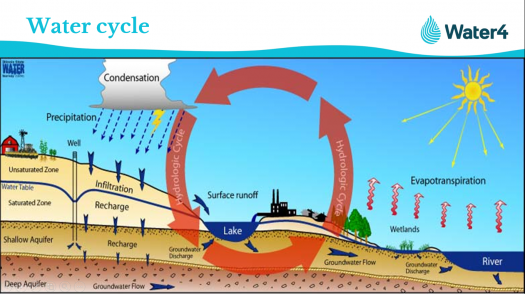# Week 2: Basic Water Cycle Quiz

14 Questions | Total Attempts: 18SettingsPAPER CODE: G. S-1011 Instructions: There is a total of 14 questions in this paper. The entire paper is divided into two sections, A and B. All sections are compulsory. Questions in each section are of different types. Section – A contains a total of 10 Multiple Choice Questions (MCQ). Each MCQ type question has four choices out of which only one choice is the correct answer. Section – B contains a total of 4 Structured and short assay questions.

• 1.
Where does the energy for the water cycle come from?
• A.

A) Moon

• B.

B) Fossil fuel

• C.

C) Sun

• D.

D) Electricity

• 2.
Which of the following is NOT a way that water moves from the land to the atmosphere?
• A.

A) Evaporation

• B.

B) Precipitation

• C.

C) Sublimation

• D.

D) Transpiration

• 3.
In what form can we see water in the atmosphere?
• A.

A) Lakes

• B.

B) Rivers

• C.

C) Oceans

• D.

D) Clouds

• 4.
The water cycle is…………………………………………………….?
• A.

A) The continuous movement of water

• B.

B) How water goes through a house

• C.

C) The stuff in water

• D.

D) All the above

• 5.
When water turns to vapor and rises into the atmosphere its called……………………….?
• A.

A) Evaporation

• B.

B) Transpiration

• C.

C) Sublimation

• D.

D) Precipitation

• 6.
95% of the earth’s water is stored in its:
• A.

A) Rivers

• B.

B) Oceans

• C.

C) Glaciers

• D.

D) Underground aquifers

• 7.
When water vapor cools into a liquid it’s called:
• A.

A) Condensation

• B.

B) Transpiration

• C.

C) Sublimation

• D.

D) Precipitation

• 8.
When water droplets get large enough to fall from the cloud it’s called:
• A.

A) Condensation

• B.

B) Transpiration

• C.

C) Sublimation

• D.

D) Precipitation

• 9.
Fresh water running underground are called:
• A.

A) Rivers

• B.

B) Streams

• C.

C) Creeks

• D.

D) Aquifers

• 10.
Water entering the ground through…………………………
• A.

A) Condensation

• B.

B) Transpiration

• C.

C) Infiltration

• D.

D) Groundwater discharge

• 11.
What is water cycle?
• 12.
How is water essential to living things
• 13.
Differentiate between infiltration and runoff?
• 14.
Describe the main stages of the water cycle?Back to top